# Worksheet Question - Simple Equations Class 7 Notes | EduRev

## Class 7 : Worksheet Question - Simple Equations Class 7 Notes | EduRev

The document Worksheet Question - Simple Equations Class 7 Notes | EduRev is a part of the Class 7 Course Mathematics (Maths) Class 7.
All you need of Class 7 at this link: Class 7

Ques 1: Solve the following equations:
(a)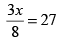(b) 5x + 3 =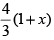(c) 0.15 (5x – 2) = 0.4 (x + 1)
Ans: (a) x = 72
(b) x =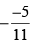(c) x = 1.8

Ques 2: Write the following equation in the form of statement:
(a)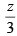+ 3 = 30
(b)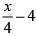= 4
Ans: (a) If you add 3 to one third of z, you get 30.
(b) One-fourth of a number x minus 4 gives 4.

Ques 3: Write equations for the following statement:
(a) The length of a rectangle is 5 more than its breadth and its perimeter is 250m.
(b) One third of a number is 8 less than the three times of the number.
Ans: (a) 2(2b + 5) = 250
(b)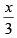= 3x - 8

Ques 4: If x = y + 2, then find the value of y in euqation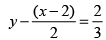Ans: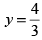Ques 5: Solve the following equations:
(a) 4 5(m 1) 34
(b) 0 = 16 + 4 (n – 6)
Ans: (a) m = 7
(b) n = 2

Ques 6: People of Anandgram planted trees in the village garden. Some of the trees were fruit trees. The number of non fruit trees were two more than three times the number of fruit trees.
(a) What was the number of fruit trees planted if the number of non-fruit trees planted was 93.
(b) Write two benefits of plantation of tree.
Ans: (a) 30 trees
(b) (1) Trees give us fruits, (any two benefits)
(2) Trees make environment pure.

Ques 7: If the difference of two complenentary angles is 10° then find measure of each angle.
Ans: 40°, 50°

Ques 8: Find the measure of such angle whose supplementary angle is 35° more than twice of its complementary angle.
Ans: 35°

Ques 9: Set up equaiton and solve it find the unknown number. “If I take three-fourth of a number and add 3 to it, I get 21.”
Ans: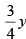+ 3 = 21, y = 24

Ques 10: The ratio of Nisha and Nishant ages in 4 : 5. After 10 years the father's age will become 5:6. Find their presant ages.
Ans: 40 year, 50 year

Ques 11: A father is 35 years more than his son's age. After 5 years the father's age will be twice of his son's age. Find the presant age of both.
Ans: 30 year, 65 year

Ques 12: Solve the following riddle : I am a number Take me seven times over Tell my identity!
Take me seven times over And add a fifty.
To reach a triple century Your shall need forty.
Ans: 30

Offer running on EduRev: Apply code STAYHOME200 to get INR 200 off on our premium plan EduRev Infinity!

## Mathematics (Maths) Class 7

209 videos|109 docs|45 tests

,

,

,

,

,

,

,

,

,

,

,

,

,

,

,

,

,

,

,

,

,

;